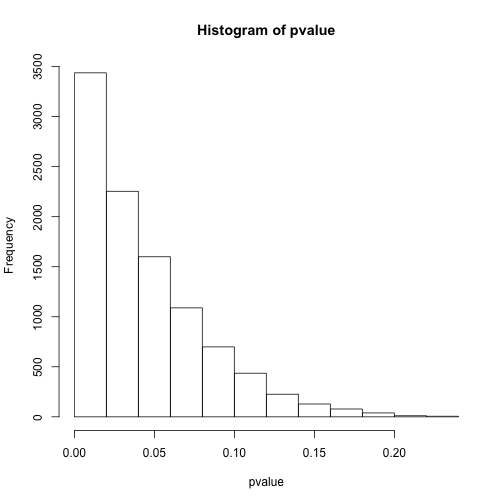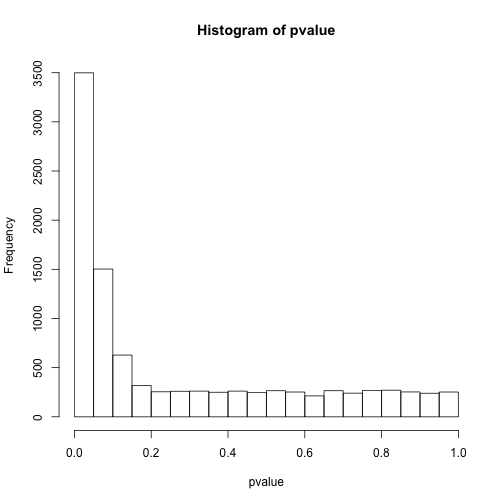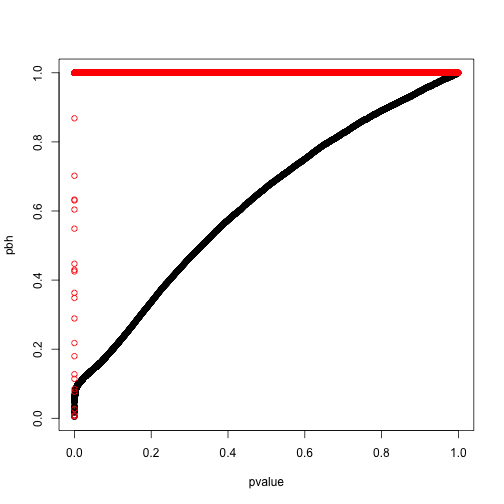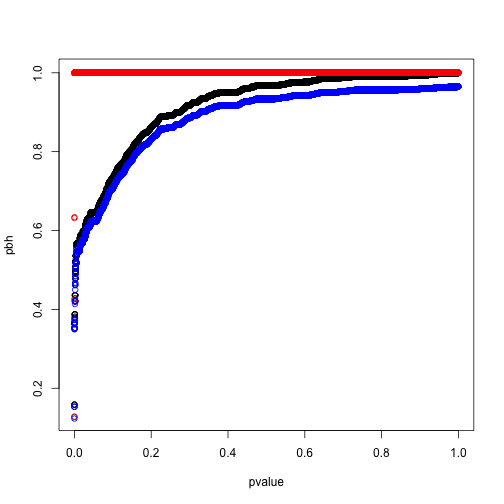4605 字

# 高通量数据的多重检验问题

## 整体错误率（Family-wise error rate）控制

# 随机数的10000次比较
set.seed(42)
pvalue <- NULL
for (i in 1:10000){
a <- rnorm(10)
b <- rnorm(10)
c <- t.test(a,b)
pvalue[i] <- c$p.value } # 看下p值分布 hist(pvalue)plot of chunk null # 小于0.05的个数 sum(pvalue<0.05) ##  477 # 小于0.0001的个数 sum(pvalue<0.0001) ##  0 这样我们会看到进行了整体的控制之后，确实是找不到有差异的了，但假如里面本来就有有差异的呢？ set.seed(42) pvalue <- NULL for (i in 1:10000){ a <- rnorm(10,1) b <- a+1 c <- t.test(a,b) pvalue[i] <- c$p.value
}
# 看下p值分布
hist(pvalue)plot of chunk diff

# 小于0.05的个数
sum(pvalue<0.05)
##  6559
# 小于0.0001的个数
sum(pvalue<0.0001)
##  45

set.seed(42)
pvalue <- NULL
for (i in 1:5000){
a <- rnorm(10,1)
b <- a+1
c <- t.test(a,b)
pvalue[i] <- c$p.value } for (i in 1:5000){ a <- rnorm(10,1) b <- rnorm(10,1) c <- t.test(a,b) pvalue[i+5000] <- c$p.value
}
# 看下p值分布
hist(pvalue)plot of chunk mix

# 小于0.05的个数
sum(pvalue<0.05)
##  3499
# 小于0.0001的个数
sum(pvalue<0.0001)
##  21

### Bonferroni 方法

$P(至少一个显著)=1-P(无显著差异) = 1-(1-\alpha/n)^n$

n <- c(1:10 %o% 10^(1:2))
p0 <- 1-(1-0.05)^n
p <- 1-(1-0.05/n)^n
# 不进行控制
plot(p0~n,ylim = c(0,1))
# Bonferroni方法控制
points(p~n,pch=19)plot of chunk Bonferroni

### Sidak 方法

$P(至少一个显著)=1-P(无显著差异) = 1-(1-\alpha')^n = 0.05$

## 错误发现率（False Discovery Rate）控制

# 所有有差异的
R <- sum(pvalue<0.05)
# 假阳性
V <- sum(pvalue[5001:10000]<0.05)
# 错误发现率
Q <- V/R
R
##  3499
V
##  225
Q
##  0.06430409

# 所有有差异的
R <- sum(pvalue<0.01)
# 假阳性
V <- sum(pvalue[5001:10000]<0.01)
# 错误发现率
Q <- V/R
R
##  999
V
##  34
Q
##  0.03403403

### Benjamini-Hochberg方法

$p_i \leq \frac{i}{m} \alpha$

pbh <- p.adjust(pvalue,method = 'BH')
plot(pbh~pvalue)
points(ph~pvalue,col='red')plot of chunk BH

### Storey方法（q值）

$\hat\pi_0 = \frac{\#\{p_i>\lambda\}}{(1-\lambda)m}$

library(qvalue)
q <- qvalue(pvalue)
# Q值
plot(q$qvalues~pvalue,col='blue')plot of chunk qvalues 如上图所示，q值增大后会最终逼近到0.5，而我们的模拟中空假设的比例就设定就是50%。我们重新模拟一个空假设比例5%的实验： set.seed(42) pvalue <- NULL for (i in 1:500){ a <- rnorm(10,1) b <- a+1 c <- t.test(a,b) pvalue[i] <- c$p.value
}
for (i in 1:9500){
a <- rnorm(10,1)
b <- rnorm(10,1)
c <- t.test(a,b)
pvalue[i+500] <- c$p.value } pbh <- p.adjust(pvalue,method = 'BH') ph <- p.adjust(pvalue,method = 'holm') q <- qvalue(pvalue) plot(pbh~pvalue) # Holm 方法 points(ph~pvalue,col='red') # Q值 points(q$qvalues~pvalue,col='blue')plot of chunk qbh